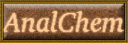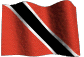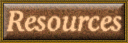# Metric Equivalents and Conversion Tables

HOME Email Webmaster

Related link:
Metric converter

# Temperature

 Degrees Celsius to Fahrenheit multiply by 1.8 (or 9/5) and add 32 Degrees Fahrenheit to Celsius subtract 32 and multiply by 0.55 (or 5/9)

# Speeds

 Mph 10 20 30 40 50 60 70 80 90 100 Kph 16 32 48 64 80 96 112 128 144 160

# To convertLength, distance & area

 Inches to centimetres, multiply by 2.54 1 in. = 2.54 cm Centimetres to inches, multiply by 0.39 1 cm = 0.3937 in Feet to metres, multiply by 0.3 1 ft = 0.3048 meter Metres to feet, multiply by 3.28 1 m =3.280 ft Yards to metres, multiply by 0.91 1 yd = 0.914 meter Metres to yards, multiply by 1.09 1 m = 1.0936 yd Miles to kilometres, multiply by 1.61 1 mile = 1.609 Km = 5280 ft = 1760 yds 1 nautical mile = 6076.2 ft = 2025.4 yds Kilometres to miles, multiply by 0.62 1 Km = 0.621 mile = 5/8 mile Acres to hectares, multiply by 0.4 1 acre = 0.405 hectare = 43,560 sq. ft. = 4,840 sq. yds. = 4,046.9 sq. meters Hectares to acres, multiply by 2.47 1 hectare =2.47 acres

# Inches to millimeters

 inch mm inch mm 1 /4 (0.250) 6.3500 11/16 (0.6875) 17.4625 3/4 (0.750) 19.0500 13/16 (0.8125) 20.6375 1/2 (0.500) 12.700 1/32 (0.0313) 0.7938 1/3 (0.33) 8.46 3/32 (0.0938) 2.3813 2/3 (0.66) 16.93 1 inch 25.4 1/8 (0.125) 3.1750 1 foot 30 cm 3/8 (0.375) 9.5250 1 meter 3.28 ft 5/8 (0.625) 15.8750 0.025 in 0.6 7/8 (0.875) 22.2250 0.049 in 1.0 1/16 (0.0625) 1.5875 0.120 in 3.0 3/16 (0.1875) 4.7625 0.170 in 4.3 5/16 (0.3125) 7.9375 7/16 (0.4375) 11.1125 9/16 (0.5625) 14.2875

# Weight

 To convert ounces to grams, multiply by 28.35 1 oz =28.349 gm To convert grams to ounces, multiply by 0.035 1 gm = 0.03527 oz Pounds to kilograms, multiply by 0.45 1 lb =0.45359 kg Kilograms to pounds, multiply by 2.21 1 kg = 2.2046 lb British ton to kilograms, multiply by 1016 1 kg = 2.2046 lb British ton to kilograms, multiply by 1016 1 ton = 1,016 kg = 2240 lbs = 1.016 tonne US tons to kilograms, multiply by 907 1 tonne = 1000 kg = 2200.6 lbs = 0.9842 ton

# Volume

 To convert imperial gallons to litres multiply by 4.55 1 imp gall = 4.546 liters = 10 lbs. To convert imperial gallons to litres multiply by 0.22 1 imp liter = 0.21996 gall US gallons to litres multiply by 3.79 1 US gall = 3.7854 liters =8.33 lbs Litres to US gallons multiply by 0.26 1 US liter = 0.26417 gall US gallons to Imp gallons multiply by 0.8333 Imp gallons to US gallons multiply by 1.2009

# Metric Equivalents

 Power-energy-force-pressure-viscosity 1 watt = 1 joule per second 1 watt = 0.738 foot pound force per sec. 1 watt = 0.057 btu/min 1 watt = 1 newton meter per sec 1 kilowatthour (KWh) = 3.6 megajoules 1 Btu = 1055.056 joules 1 ft.lb/s2 = 0.138kg.m/s2 1 kg.ms2 = 1 newton 1 atmosphere = 101.325 kilopascals (kPa) 1 atmosphere = 1.0133 bars 1 atmosphere = 14.70 psi 1 atmosphere = 760mm Hg 1 newton per sq.m. = 1 pascal 1 centipoise = 1 centistoke x density 1 centistoke = 1 x 10-6m2/s 1 centistoke = 1 x 10-3 pascal-seconds

# Common Conversion Factors

Quantity SI Unit Other Unit Conversion Factor
Energy joule calorie
erg
1 cal = 4.184 J
1 erg = 10-7 J
Force newton dyne 1 dyn = 10-5 N
Length metre ångström 1 Å = 10-10 m = 10-8 cm = 10-1 nm
Mass kilogram pound 1 lb = 0.453592 kg
Pressure pascal bar
atmosphere
mm Hg
lb/in2
1 bar = 105 Pa
1 atm = 1.01325 x 105 Pa
1 mm Hg = 133.322 Pa
1 lb/in2 = 6894.8 Pa
Temperature kelvin Celsius
Fahrenheit
1°C = 1 K
1°F = 5/9 K
Volume cubic metre litre
gallon (U.S.)
gallon (U.K.)
cubic inch
1 L = 1 dm3 = 10-3 m3
1 gal (U.S.) = 3.7854 x 10-3 m3
1 gal (U.K.) = 4.5641 x 10-3 m3
1 in3 = 1.6387 x 10-6 m3

 Capacity(US) Linear dimensional Mass 1 ml = 0.034 oz 1 oz = 29.573 ml 1 ml = 0.270 dram 1 dram = 3.697 ml 1 liter = 1.07 quarts 1 quart = 0.946 liter 1 liter = 0.264 gallon 1 gallon = 3.785 liters 1 cm = 0.394 in 1 inch = 2.540 cm 1 meter = 3.281 ft 1 ft = 0.305 m 1 meter = 1.094 yds 1 yard = 0.914 meter 1 kilometer = 0.621 mile 1 kilometer = 5/8 mile 1 mile = 1.609 km 1 gram = 15.432 grains 1 grain = 0.065 gram 1 gram = 0.035 oz 1 oz = 28.349 gram 1 kilogram = 2.205 lb 1 lb = 0.454 kg

# Specific Conversion Factor Tables Volume

Multiply the left column by the middle column to get values for right column
and divide right column by middle column to get values for left column

 Multiply → To get To get ← Divide Cubic cm (cc) Oz (fluid) Cubic ft. Cubic ft. Gal Gal Gal Cubic meters 1 29.57 7.48 0.0283 128 3.785 0.8333 1000 ml. ml. ml. m3 Oz (fluid) Liters Imp. Gal. liters

# Conversion Tables Horsepower / Watts

Multiply the left column by the middle column to get values for right column
and divide the right column by the middle column to get values for left column

 Multiply → To get To get ← Divide Hp Hp Hp Hp Watts Watts lb-ft. oz in. oz-in. oz-in. Gauss 0.746 42.44 396000 1.014 0.7376 44.25 0.1368 0.072 70,6000 0.00706 0.0001 Kw Btu / min. lb.in / min. metric hp lb ft, / sec. lb ft, / min. kg-m kg-cm. dyne-cm. Nm. Tesla

# Conversion Tables Pressure / Vacuum

Multiply the left column by the middle column to get values for right column
and divide the right column by middle column to get values for left column

 Multiply → To get To get ← Divide Atm Atm Atm Atm Atm Atm Bar Bar Bar ft. H2O ft. H2O in. Hg in. Hg in. Hg kPa KPa KPa Psi 33.9 760 1033.2 14.70 1.013 101.3 14.5 0.9869 100 0.4335 0.8826 0.4912 25.4 3.386 0.01 0.1450 7.5 0.0703 ft. H2O mm Hg g / cm2 Psi Bar KPa Psi Atm KPa Psi in. Hg psi mm Hg kPa dyne / cm2 Psi mm Hg kg/ cm 3

# Conversion Tables Flowrate

 Multiply → To get To get ← Divide cc / min. cfm (ft3 / min) cfm cfh (ft3 / hr) cfh GPH GPH GPM GPM oz / min. 1 28.31 1.699 472 0.125 63.1 0.134 0.227 3.785 29.57 ml / min L / min m3 / hr ml / min GPM ml / min cfh m3 / hr L / min ml / min

# Conversion Tables Mass

Multiply the left column by the middle column to get values for right column
and divide the right column by middle column to get values for left column

 Multiply → To get To get ← Divide dram dram dram dram 60 0.1371 0.008571 3.88794 grains oz lb grams lb lb lb lb 5760 13.1657 0.822857 373.242 grains oz lb grams oz oz oz oz 480 1.09714 0.068571 31.1035 grains oz lb grams drams drams drams drams 27.344 0.0625 0.003906 1.77185 grains oz lb grams lb lb lb oz 7000 16 453.592 437.5 grains oz grams grains oz oz carats carats 0.0625 28.3495 3.0865 0.2 lb grams grains grams grams grams lb oz 15.432 0.0353 0.4536 28.35 grains oz Kg grams

Signature: Dhanlal De Lloyd, Chem. Dept, The University of The West Indies, St. Augustine campus
The Republic of Trinidad and Tobago.
Copyright: delloyd2000© All rights reserved.